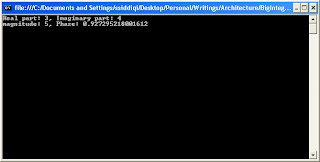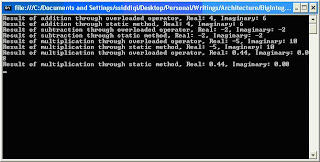## Monday, May 31, 2010

### Complex Numbers in .net 4.0

Today we are going to discuss about a new type introduced in .net 4.0. This type is Complex. This is a struct type, and is available in System.Numerics namespace. Writing engineering and scientific applications, we encounter complex numbers. Since this was not provided by the framework, we always had to implement in code. Let us start with complex numbers.

As we know that complex numbers can be represented in Cartesian (a, b) and polar coordinates (r, Θ). This number is generally written as follows in scientific calculations:

a + bi

Or in electrical engineering as:

a + jb

Here i or j represents the imaginary part of complex number. As you might know, Euler named it as “imaginary unity”.

The good thing is that the framework supports both representations. Let us start with initialization of complex numbers in both these representations. But before that you would have to add reference of System.Numerics library.Complex num = new Complex(2, 3);

Complex num2 = Complex.FromPolarCoordinates(5, 0.5);

As you can see that, to declare complex number using polar coordinates, we have to use the static method FromPolarCoordinates().As we know the following:

a = r CosΘ

b = r SinΘ

Let us write our first piece of code involving complex numbers. In the following code, a complex number is initiated with 3 as its real and 4 as its imaginary part. Then magnitude and phase are printed to see if they have been correctly evaluated.

Complex num = new Complex(3, 4);

Console.WriteLine("Real part: {0}, Imaginary part: {1}", num.Real, num.Imaginary);

Console.WriteLine("magnitude: {0}, Phase: {1}", num.Magnitude, num.Phase);Let us do an exercise of instantiating using polar representation. This results in the same output as earlier.

Complex num2 = Complex.FromPolarCoordinates(5, 0.927295218001612);

Console.WriteLine("Real part: {0}, Imaginary part: {1}", num2.Real, num2.Imaginary);

Console.WriteLine("magnitude: {0}, Phase: {1}", num2.Magnitude, num2.Phase);

Now let us apply some basic arithmetic operations to complex numbers. These arithmetic operations might be applied using the overloaded operators or static method defined in Complex structure.

Complex num1 = new Complex(1, 2);

Complex num2 = new Complex(3, 4);

Complex numSubtractThroughOverloadedOperator = num1 - num2;

Complex numSubtractThroughStaticMethod = Complex.Subtract(num1, num2);

Console.WriteLine("Result of subtraction through static method, Real: {0}, Imaginary: {1}", numSubtractThroughStaticMethod.Real, numSubtractThroughStaticMethod.Imaginary);

Complex numMultiplicationThroughOverloadedOperator = num1 * num2;

Complex numMultiplicationThroughStaticMethod = Complex.Multiply(num1, num2);

Console.WriteLine("Result of multiplication through static method, Real: {0}, Imaginary: {1}", numMultiplicationThroughStaticMethod.Real, numMultiplicationThroughStaticMethod.Imaginary);

Complex numDivisionThroughOverloadedOperator = num1 / num2;

Complex numDivisionThroughStaticMethod = Complex.Divide(num1, num2);

Console.WriteLine("Result of multiplication through static method, Real: {0}, Imaginary: {1}", numDivisionThroughStaticMethod.Real, numDivisionThroughStaticMethod.Imaginary);Let’s verify one of the above calculations. We select multiplication operations:

(1, 2) * (3, 4) = (1 + j2) * (3 + j4)

= 3 + j4 + j6 + (j * j) 8

= 3 + j10 -8 = -5 + j10

Complex numbers might also be compared. Both Equality (= =) and Inequality (!=) operators are overloaded. Additionally, Equals method is overridden. There are basically two versions of Equals(). One is the general one which is available with all .net types (System.Object). The other one is available because Complex structure implements the IEquatable interface.

Complex num1 = new Complex(1, 2);

Complex num2 = new Complex(1, 2);

if (num1 == num2)

Console.WriteLine("num1 == num2 resulted in true condition");

else

Console.WriteLine("num1 == num2 resulted in false condition");

if (num1.Equals(num2))

Console.WriteLine("num1.Equals(num2) resulted in true condition");

else

Console.WriteLine("num1.Equals(num2) resulted in false condition");

This results in the following output:Complex structure also supports trigonometric functions including regular ones like Sine, Cosine etc. It also supports their inverses, hyperbolics and inverse hyperbolics.

Complex num = new Complex(3, 4);

Complex sinComplex = Complex.Sin(num);

Console.WriteLine("Result of Sin on Complex number, Real: {0}, Imaginary: {1}", sinComplex.Real, sinComplex.Imaginary);

This would write the Sine value of the specified complex number in the exact format.

We have the following formula for Cosine hyperbolics of a complex number. Let us verify if we could get the same result from the static method available in Complex structure.Complex num = new Complex(3, 4);

Complex cosh_Direct = Complex.Cosh(num);

Console.WriteLine("Result of Sin on Complex number, Real: {0}, Imaginary: {1}", cosh_Direct.Real, cosh_Direct.Imaginary);

Complex Cosh_Calculated = new Complex(Math.Cosh(num.Real) * Math.Cos(num.Imaginary), Math.Sinh(num.Real) * Math.Sin(num.Imaginary));

if (cosh_Direct.Equals(Cosh_Calculated))

Console.WriteLine("Cosine hyperbolic correctly calculated");

else

Console.WriteLine("Cosine hyperbolic incorrectly calculated");

As we predicted, this prints out “Cosine hyperbolic correctly calculated” on the screen.

There are other features provided too like taking conjugate, inverse, logarithm etc. of a complex number. We would not be going in the details about all of them just to save some length of this discussion.